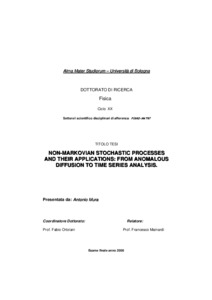# Non-Markovian stochastic processes and their applications: from anomalous diffusion to time series analysis

Mura, Antonio (2008) Non-Markovian stochastic processes and their applications: from anomalous diffusion to time series analysis, [Dissertation thesis], Alma Mater Studiorum Università di Bologna. Dottorato di ricerca in Fisica, 20 Ciclo. DOI 10.6092/unibo/amsdottorato/846.
Documenti full-text disponibili:Anteprima
Documento PDF (English) - Richiede un lettore di PDF come Xpdf o Adobe Acrobat Reader

## Abstract

This work provides a forward step in the study and comprehension of the relationships between stochastic processes and a certain class of integral-partial differential equation, which can be used in order to model anomalous diffusion and transport in statistical physics. In the first part, we brought the reader through the fundamental notions of probability and stochastic processes, stochastic integration and stochastic differential equations as well. In particular, within the study of H-sssi processes, we focused on fractional Brownian motion (fBm) and its discrete-time increment process, the fractional Gaussian noise (fGn), which provide examples of non-Markovian Gaussian processes. The fGn, together with stationary FARIMA processes, is widely used in the modeling and estimation of long-memory, or long-range dependence (LRD). Time series manifesting long-range dependence, are often observed in nature especially in physics, meteorology, climatology, but also in hydrology, geophysics, economy and many others. We deepely studied LRD, giving many real data examples, providing statistical analysis and introducing parametric methods of estimation. Then, we introduced the theory of fractional integrals and derivatives, which indeed turns out to be very appropriate for studying and modeling systems with long-memory properties. After having introduced the basics concepts, we provided many examples and applications. For instance, we investigated the relaxation equation with distributed order time-fractional derivatives, which describes models characterized by a strong memory component and can be used to model relaxation in complex systems, which deviates from the classical exponential Debye pattern. Then, we focused in the study of generalizations of the standard diffusion equation, by passing through the preliminary study of the fractional forward drift equation. Such generalizations have been obtained by using fractional integrals and derivatives of distributed orders. In order to find a connection between the anomalous diffusion described by these equations and the long-range dependence, we introduced and studied the generalized grey Brownian motion (ggBm), which is actually a parametric class of H-sssi processes, which have indeed marginal probability density function evolving in time according to a partial integro-differential equation of fractional type. The ggBm is of course Non-Markovian. All around the work, we have remarked many times that, starting from a master equation of a probability density function f(x,t), it is always possible to define an equivalence class of stochastic processes with the same marginal density function f(x,t). All these processes provide suitable stochastic models for the starting equation. Studying the ggBm, we just focused on a subclass made up of processes with stationary increments. The ggBm has been defined canonically in the so called grey noise space. However, we have been able to provide a characterization notwithstanding the underline probability space. We also pointed out that that the generalized grey Brownian motion is a direct generalization of a Gaussian process and in particular it generalizes Brownain motion and fractional Brownain motion as well. Finally, we introduced and analyzed a more general class of diffusion type equations related to certain non-Markovian stochastic processes. We started from the forward drift equation, which have been made non-local in time by the introduction of a suitable chosen memory kernel K(t). The resulting non-Markovian equation has been interpreted in a natural way as the evolution equation of the marginal density function of a random time process l(t). We then consider the subordinated process Y(t)=X(l(t)) where X(t) is a Markovian diffusion. The corresponding time-evolution of the marginal density function of Y(t) is governed by a non-Markovian Fokker-Planck equation which involves the same memory kernel K(t). We developed several applications and derived the exact solutions. Moreover, we considered different stochastic models for the given equations, providing path simulations.

Abstract
Tipologia del documento
Tesi di dottorato
Autore
Mura, Antonio
Supervisore
Dottorato di ricerca
Ciclo
20
Coordinatore
Settore disciplinare
Parole chiave
non-markovian stochastic processes long-range dependence anomalous diffusion fractional calculus grey brownian motion fractional brownian motion
URN:NBN
DOI
10.6092/unibo/amsdottorato/846
Data di discussione
12 Giugno 2008
URI